# 7.6 Finding the equation of a line  (Page 2/2)

 Page 2 / 2

Notice that both forms rely on knowing the slope. If we are given two points on the line we may still find the equation of the line passing through them by first finding the slope of the line, then using the point-slope form.

It is customary to use either the slope-intercept form or the general form for the final form of the line. We will use the slope-intercept form as the final form.

## Sample set a

Find the equation of the line using the given information.

$m=6 ,\text{}\text{}\text{}\text{}y\text{-intercept\hspace{0.17em}}\text{}\left(0,4\right)$

Since we’re given the slope and the $y\text{-intercept,}$ we’ll use the slope-intercept form. $m=6,b=4.$

$\begin{array}{l}y=mx+b\hfill \\ y=6x+4\hfill \end{array}$

$m=-\frac{3}{4}, \text{}\text{}\text{}\text{}y\text{-intercept\hspace{0.17em}}\text{}\left(0,\frac{1}{8}\right)$

Since we’re given the slope and the $y\text{-intercept,}$ we’ll use the slope-intercept form. $m=\frac{-3}{4},$

$\begin{array}{ccc}b& =& \frac{1}{8}.\\ y& =& mx+b\\ y& =& -\frac{3}{4}x+\frac{1}{8}\end{array}$

$\begin{array}{cc}m=2,& \text{the\hspace{0.17em}}\text{}\text{point}\left(4,3\right).\end{array}$
Write the equation in slope-intercept form.

Since we’re given the slope and some point, we’ll use the point-slope form.

$\begin{array}{llll}y-{y}_{1}\hfill & =\hfill & m\left(x-{x}_{1}\right)\hfill & \text{Let\hspace{0.17em}}\text{}\left({x}_{1},{y}_{1}\right) \text{}\text{be\hspace{0.17em}}\text{}\text{(4,3)}\text{.}\hfill \\ y-3\hfill & =\hfill & 2\left(x-4\right)\hfill & \text{Put\hspace{0.17em}}\text{}\text{this\hspace{0.17em}}\text{}\text{equation\hspace{0.17em}}\text{}\text{in\hspace{0.17em}}\text{}\text{slope-intercept\hspace{0.17em}}\text{}\text{form\hspace{0.17em}}\text{}\text{by\hspace{0.17em}}\text{}\text{solving\hspace{0.17em}}\text{}\text{for\hspace{0.17em}}\text{}y.\hfill \\ y-3\hfill & =\hfill & 2x-8\hfill & \hfill \\ \hfill y& =\hfill & 2x-5\hfill & \hfill \end{array}$

$\begin{array}{cc}m=-5,& \text{the\hspace{0.17em}}\text{}\text{point}\left(-3,0\right).\end{array}$
Write the equation in slope-intercept form.

Since we’re given the slope and some point, we’ll use the point-slope form.

$\begin{array}{llll}y-{y}_{1}\hfill & =\hfill & m\left(x-{x}_{1}\right)\hfill & \text{Let\hspace{0.17em}}\text{}\left({x}_{1},{y}_{1}\right) \text{}\text{be\hspace{0.17em}}\text{}\text{(-3,0)}\text{.}\hfill \\ y-0\hfill & =\hfill & -5\left[x-\left(-3\right)\right]\hfill & \hfill \\ \hfill y& =\hfill & -5\left(x+3\right)\hfill & \text{Solve\hspace{0.17em}}\text{}\text{for\hspace{0.17em}}\text{}y.\hfill \\ \hfill y& =\hfill & -5x-15\hfill & \hfill \end{array}$

$\begin{array}{cc}m=-1,& \text{the\hspace{0.17em}}\text{}\text{point}\left(0,7\right).\end{array}$
Write the equation in slope-intercept form.

We’re given the slope and a point, but careful observation reveals that this point is actually the $y\text{-intercept}\text{.}$ Thus, we’ll use the slope-intercept form. If we had not seen that this point was the $y\text{-intercept}$ we would have proceeded with the point-slope form. This would create slightly more work, but still give the same result.

$\begin{array}{ll}\underset{¯}{\text{Slope-intercept\hspace{0.17em}}\text{}\text{form}}\hfill & \underset{¯}{\text{Point-slope\hspace{0.17em}}\text{}\text{form}}\hfill \\ \begin{array}{lll}y\hfill & =\hfill & mx+b\hfill \\ y\hfill & =\hfill & -1x+7\hfill \\ y\hfill & =\hfill & -x+7\hfill \\ \hfill & \hfill & \hfill \end{array}\hfill & \begin{array}{lll}y-{y}_{1}\hfill & =\hfill & m\left(x-{x}_{1}\right)\hfill \\ y-7\hfill & =\hfill & -1\left(x-0\right)\hfill \\ y-7\hfill & =\hfill & -x\hfill \\ y\hfill & =\hfill & -x+7\hfill \end{array}\hfill \end{array}$

The two points $\left(4,1\right)$ and $\left(3,5\right).$
Write the equation in slope-intercept form.

Since we’re given two points, we’ll find the slope first.

$m=\frac{{y}_{2}-{y}_{1}}{{x}_{2}-{x}_{1}}=\frac{5-1}{3-4}=\frac{4}{-1}=-4$

Now, we have the slope and two points. We can use either point and the point-slope form.

 Using $\left(4, \text{}1\right)$ Using $\left(3, \text{}5\right)$ $\begin{array}{lll}y-{y}_{1}\hfill & =\hfill & m\left(x-{x}_{1}\right)\hfill \\ y-1\hfill & =\hfill & -4\left(x-4\right)\hfill \\ y-1\hfill & =\hfill & -4x+16\hfill \\ y\hfill & =\hfill & -4x+17\hfill \end{array}$ $\begin{array}{lll}y-{y}_{1}\hfill & =\hfill & m\left(x-{x}_{1}\right)\hfill \\ y-5\hfill & =\hfill & -4\left(x-3\right)\hfill \\ y-5\hfill & =\hfill & -4x+12\hfill \\ y\hfill & =\hfill & -4x+17\hfill \end{array}$

We can see that the use of either point gives the same result.

## Practice set a

Find the equation of each line given the following information. Use the slope-intercept form as the final form of the equation.

$m=5, \text{}y\text{-intercept\hspace{0.17em}}\text{}\left(0,8\right).$

$y=5x+8$

$m=-8, \text{}y\text{-intercept\hspace{0.17em}}\text{}\left(0,3\right).$

$y=-8x+3$

$m=2, \text{}y\text{-intercept\hspace{0.17em}}\text{}\left(0,-7\right).$

$y=2x-7$

$m=1, \text{}y\text{-intercept\hspace{0.17em}}\text{}\left(0,-1\right).$

$y=x-1$

$m=-1, \text{}y\text{-intercept\hspace{0.17em}}\text{}\left(0,-10\right).$

$y=-x-10$

$m=4,$ the point $\left(5,2\right).$

$y=4x-18$

$m=-6,$ the point $\left(-1,0\right).$

$y=-6x-6$

$m=-1,$ the point $\left(-5,-5\right).$

$y=-x-10$

The two points $\left(4,1\right)$ and $\left(6,5\right).$

$y=2x-7$

The two points $\left(-7,-1\right)$ and $\left(-4,8\right).$

$y=3x+20$

## Sample set b

Find the equation of the line passing through the point $\left(4,-7\right)$ having slope 0.

We’re given the slope and some point, so we’ll use the point-slope form. With $m=0$ and $\left({x}_{1},{y}_{1}\right)$ as $\left(4,-7\right),$ we have

$\begin{array}{lll}y-{y}_{1}\hfill & =\hfill & m\left(x-{x}_{1}\right)\hfill \\ y-\left(-7\right)\hfill & =\hfill & 0\left(x-4\right)\hfill \\ y+7\hfill & =\hfill & 0\hfill \\ y\hfill & =\hfill & -7\hfill \end{array}$

This is a horizontal line.

Find the equation of the line passing through the point $\left(1,3\right)$ given that the line is vertical.

Since the line is vertical, the slope does not exist. Thus, we cannot use either the slope-intercept form or the point-slope form. We must recall what we know about vertical lines. The equation of this line is simply $x=1.$

## Practice set b

Find the equation of the line passing through the point $\left(-2,9\right)$ having slope 0.

$y=9$

Find the equation of the line passing through the point $\left(-1,6\right)$ given that the line is vertical.

$x=-1$

## Sample set c

Reading only from the graph, determine the equation of the line.

The slope of the line is $\frac{2}{3},$ and the line crosses the $y\text{-axis}$ at the point $\left(0,-3\right).$ Using the slope-intercept form we get

$y=\frac{2}{3}x-3$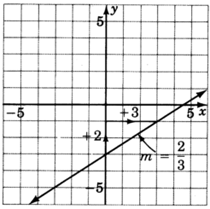## Practice set c

Reading only from the graph, determine the equation of the line.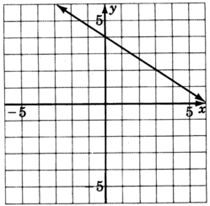$y=\frac{-2}{3}x+4$

## Exercises

For the following problems, write the equation of the line using the given information in slope-intercept form.

$m=3, \text{}y\text{-intercept\hspace{0.17em}}\text{}\left(0,4\right)$

$y=3x+4$

$m=2, \text{}y\text{-intercept\hspace{0.17em}}\text{}\left(0,5\right)$

$m=8, \text{}y\text{-intercept\hspace{0.17em}}\text{}\left(0,1\right)$

$y=8x+1$

$m=5, \text{}y\text{-intercept\hspace{0.17em}}\text{}\left(0,-3\right)$

$m=-6, \text{}y\text{-intercept\hspace{0.17em}}\text{}\left(0,-1\right)$

$y=-6x-1$

$m=-4, \text{}y\text{-intercept\hspace{0.17em}}\text{}\left(0,0\right)$

$m=-\frac{3}{2}, \text{}y\text{-intercept\hspace{0.17em}}\text{}\left(0,0\right)$

$y=-\frac{3}{2}x$

$m=3, \text{}\left(1,4\right)$

$m=1, \text{}\left(3,8\right)$

$y=x+5$

$m=2, \text{}\left(1,4\right)$

$m=8, \text{}\left(4,0\right)$

$y=8x-32$

$m=-3, \text{}\left(3,0\right)$

$m=-1, \text{}\left(6,0\right)$

$y=-x+6$

$m=-6, \text{}\left(0,0\right)$

$m=-2, \text{}\left(0,1\right)$

$y=-2x+1$

$\left(0,0\right), \text{}\left(3,2\right)$

$\left(0,0\right), \text{}\left(5,8\right)$

$y=\frac{8}{5}x$

$\left(4,1\right), \text{}\left(6,3\right)$

$\left(2,5\right), \text{}\left(1,4\right)$

$y=x+3$

$\left(5,-3\right), \text{}\left(6,2\right)$

$\left(2,3\right), \text{}\left(5,3\right)$

$y=3 \text{}\left(\text{horizontal\hspace{0.17em}}\text{}\text{line}\right)$

$\left(-1,5\right), \text{}\left(4,5\right)$

$\left(4,1\right), \text{}\left(4,2\right)$

$x=4 \text{}\left(\text{vertical\hspace{0.17em}}\text{}\text{line}\right)$

$\left(2,7\right), \text{}\left(2,8\right)$

$\left(3,3\right), \text{}\left(5,5\right)$

$y=x$

$\left(0,0\right), \text{}\left(1,1\right)$

$\left(-2,4\right), \text{}\left(3,-5\right)$

$y=-\frac{9}{5}x+\frac{2}{5}$

$\left(1,6\right), \text{}\left(-1,-6\right)$

$\left(14,12\right), \text{}\left(-9,-11\right)$

$y=x-2$

$\left(0,-4\right), \text{}\left(5,0\right)$

For the following problems, read only from the graph and determine the equation of the lines.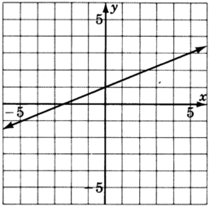$y=\frac{2}{5}x+1$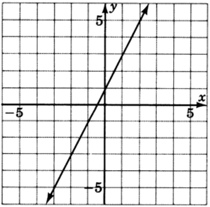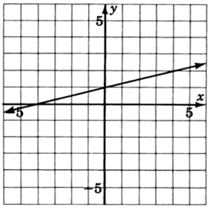$y=\frac{1}{4}x+1$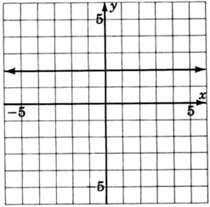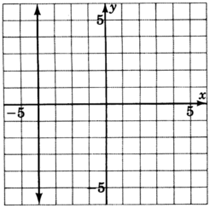$x=-4$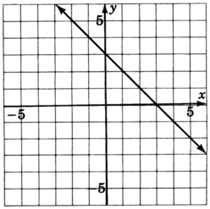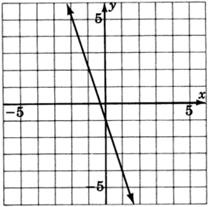$y=-3x-1$

## Exercises for review

( [link] ) Graph the equation $x-3=0.$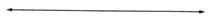( [link] ) Supply the missing word. The point at which a line crosses the $y\text{-axis}$ is called the .

$y\text{-intercept}$

( [link] ) Supply the missing word. The of a line is a measure of the steepness of the line.

( [link] ) Find the slope of the line that passes through the points $\left(4,0\right)$ and $\left(-2,-6\right).$

$m=1$

( [link] ) Graph the equation $3y=2x+3.$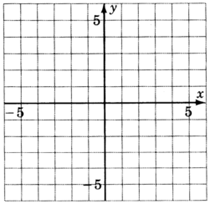how can chip be made from sand
are nano particles real
yeah
Joseph
Hello, if I study Physics teacher in bachelor, can I study Nanotechnology in master?
no can't
Lohitha
where we get a research paper on Nano chemistry....?
nanopartical of organic/inorganic / physical chemistry , pdf / thesis / review
Ali
what are the products of Nano chemistry?
There are lots of products of nano chemistry... Like nano coatings.....carbon fiber.. And lots of others..
learn
Even nanotechnology is pretty much all about chemistry... Its the chemistry on quantum or atomic level
learn
da
no nanotechnology is also a part of physics and maths it requires angle formulas and some pressure regarding concepts
Bhagvanji
hey
Giriraj
Preparation and Applications of Nanomaterial for Drug Delivery
revolt
da
Application of nanotechnology in medicine
has a lot of application modern world
Kamaluddeen
yes
narayan
what is variations in raman spectra for nanomaterials
ya I also want to know the raman spectra
Bhagvanji
I only see partial conversation and what's the question here!
what about nanotechnology for water purification
please someone correct me if I'm wrong but I think one can use nanoparticles, specially silver nanoparticles for water treatment.
Damian
yes that's correct
Professor
I think
Professor
Nasa has use it in the 60's, copper as water purification in the moon travel.
Alexandre
nanocopper obvius
Alexandre
what is the stm
is there industrial application of fullrenes. What is the method to prepare fullrene on large scale.?
Rafiq
industrial application...? mmm I think on the medical side as drug carrier, but you should go deeper on your research, I may be wrong
Damian
How we are making nano material?
what is a peer
What is meant by 'nano scale'?
What is STMs full form?
LITNING
scanning tunneling microscope
Sahil
how nano science is used for hydrophobicity
Santosh
Do u think that Graphene and Fullrene fiber can be used to make Air Plane body structure the lightest and strongest. Rafiq
Rafiq
what is differents between GO and RGO?
Mahi
what is simplest way to understand the applications of nano robots used to detect the cancer affected cell of human body.? How this robot is carried to required site of body cell.? what will be the carrier material and how can be detected that correct delivery of drug is done Rafiq
Rafiq
if virus is killing to make ARTIFICIAL DNA OF GRAPHENE FOR KILLED THE VIRUS .THIS IS OUR ASSUMPTION
Anam
analytical skills graphene is prepared to kill any type viruses .
Anam
Any one who tell me about Preparation and application of Nanomaterial for drug Delivery
Hafiz
what is Nano technology ?
write examples of Nano molecule?
Bob
The nanotechnology is as new science, to scale nanometric
brayan
nanotechnology is the study, desing, synthesis, manipulation and application of materials and functional systems through control of matter at nanoscale
Damian
how did you get the value of 2000N.What calculations are needed to arrive at it
Privacy Information Security Software Version 1.1a
Good
Please keep in mind that it's not allowed to promote any social groups (whatsapp, facebook, etc...), exchange phone numbers, email addresses or ask for personal information on QuizOver's platform.

#### Get Jobilize Job Search Mobile App in your pocket Now!ByByBy OpenStaxBy OpenStaxBy John GabrieliBy OpenStaxBy David CoreyBy Anh DaoBy Jonathan LongBy Yasser IbrahimBy Mary MateraBy OpenStax